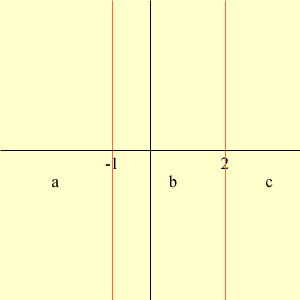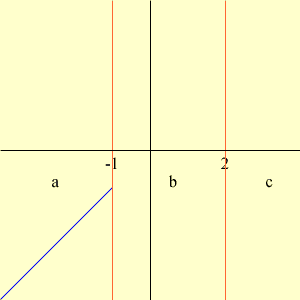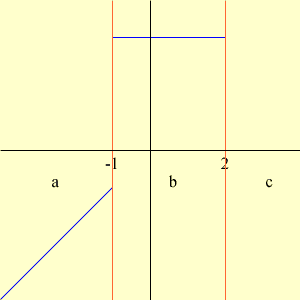Quandaries and Queries hi, my name is amber and i have talked to various tutors and teachers tonight on the internet and nobody can seem to answer my question. how do i begin to graph a piecewise function, absolute function or step function? this is an algebra II, 12 grade homework assignment.  thank you. i appreciate your help. Hi Amber, Lets look at a particular example. Suppose that you have the piecewise function defined by f(x) = x if x < -1 f(x) = 3 if -1 ≤ x ≤ 2 f(x) = -x if x > 2 To begin to graph the function, divide the x-axis into three pieces. everything less than -1 everything between -1 and 2 everything greater than 2Now, hopefully you know what the graphs the three expressions look like, f(x) = x, f(x) = 3 and f(x) = -x. f(x) = x is a straight line through the origin with slope 1. But you only need the part of the graph with x < -1. Thus that piece of the graph isf(x) = 3 is a horizontal line, three units above the x-axis. I need this graph for x between -1 and 2. Thus adding this piece of the graph I getFinally f(x) = -x is a straight line thtough the origin with slope -1. I need this for x larger than 2 and hence I getThus the graph of the piecewise function is in blue above. Notice that the way the function is defined, f(-1) = f(2) = 3 and hence I have put large dots at (-1,3) and (2,3) to emphasize this. I hope this helps, Penny Go to Math Central